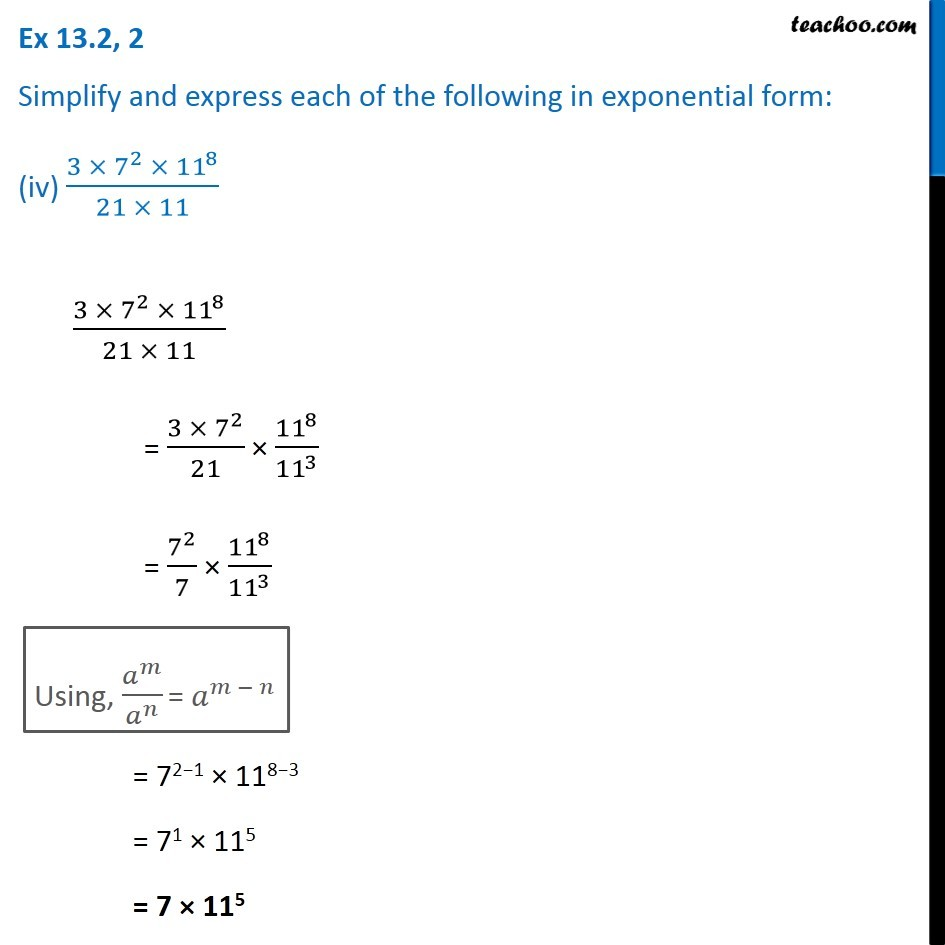1. Chapter 13 Class 7 Exponents and Powers
2. Serial order wise
3. Ex 13.2

Transcript

Ex 13.2, 2 Simplify and express each of the following in exponential form: (iv) (3 ×〖 7〗^2 ×〖 11〗^8)/(21 × 11) (3 ×〖 7〗^2 ×〖 11〗^8)/(21 × 11) = (3 ×〖 7〗^2)/21 × 〖11〗^8/〖11〗^3 = 7^2/7 × 〖11〗^8/〖11〗^3 Using, 𝑎^𝑚/𝑎^𝑛 = 𝑎^(𝑚 − 𝑛) = 72−1 × 118−3 = 71 × 115 = 7 × 115